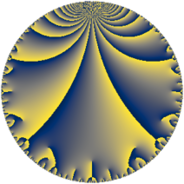Properties

 Label 29.6.bLevel $29$ Weight $6$ Character orbit 29.b Rep. character $\chi_{29}(28,\cdot)$ Character field $\Q$ Dimension $12$ Newform subspaces $1$ Sturm bound $15$ Trace bound $0$

Related objects

Defining parameters

 Level: $$N$$ $$=$$ $$29$$ Weight: $$k$$ $$=$$ $$6$$ Character orbit: $$[\chi]$$ $$=$$ 29.b (of order $$2$$ and degree $$1$$) Character conductor: $$\operatorname{cond}(\chi)$$ $$=$$ $$29$$ Character field: $$\Q$$ Newform subspaces: $$1$$ Sturm bound: $$15$$ Trace bound: $$0$$

Dimensions

The following table gives the dimensions of various subspaces of $$M_{6}(29, [\chi])$$.

Total New Old
Modular forms 14 14 0
Cusp forms 12 12 0
Eisenstein series 2 2 0

Trace form

 $$12 q - 172 q^{4} + 46 q^{5} + 24 q^{6} + 20 q^{7} - 1574 q^{9} + O(q^{10})$$ $$12 q - 172 q^{4} + 46 q^{5} + 24 q^{6} + 20 q^{7} - 1574 q^{9} + 1362 q^{13} + 340 q^{16} - 4508 q^{20} + 11376 q^{22} + 5852 q^{23} - 6292 q^{24} + 12678 q^{25} - 25056 q^{28} + 11328 q^{29} + 14952 q^{30} - 22694 q^{33} - 22504 q^{34} + 4532 q^{35} + 22840 q^{36} - 43408 q^{38} + 8280 q^{42} - 52816 q^{45} + 102836 q^{49} + 58540 q^{51} + 15172 q^{52} + 25650 q^{53} - 89080 q^{54} - 32824 q^{57} + 4960 q^{58} - 3900 q^{59} + 37720 q^{62} - 146616 q^{63} + 252276 q^{64} + 169574 q^{65} - 28264 q^{67} - 286832 q^{71} - 263072 q^{74} + 519072 q^{78} - 230964 q^{80} - 24084 q^{81} - 178008 q^{82} + 85692 q^{83} - 126624 q^{86} - 137716 q^{87} - 83604 q^{88} - 182372 q^{91} - 5664 q^{92} + 377966 q^{93} + 192144 q^{94} - 415284 q^{96} + O(q^{100})$$

Decomposition of $$S_{6}^{\mathrm{new}}(29, [\chi])$$ into newform subspaces

Label Dim. $$A$$ Field CM Traces $q$-expansion
$a_{2}$ $a_{3}$ $a_{5}$ $a_{7}$
29.6.b.a $12$ $4.651$ $$\mathbb{Q}[x]/(x^{12} + \cdots)$$ None $$0$$ $$0$$ $$46$$ $$20$$ $$q+\beta _{1}q^{2}-\beta _{4}q^{3}+(-14+\beta _{2})q^{4}+\cdots$$